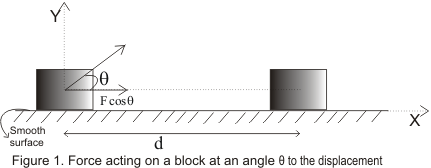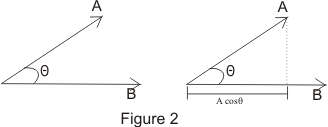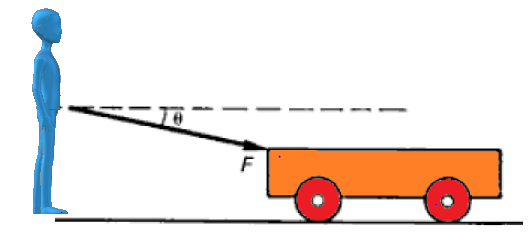# Work Done Formula and Calculation

## (1) Introduction to Work Done

• In our everyday life we use terms like work and energy.
• Term work is generally used in context to any kind of activity requiring physical or mental effort.
• But this is not the way how we define work done in physics.
• When we push or pull a heavy load or lift it above the floor then we are doing work, but a man carrying heavy load and standing still is not doing any work according to scientific definition of work.
• Another term we often use is energy. Energy is usually associated with work done in the sense that a person feeling very energetic is capable of doing lot of work.
• This way energy defined to be as capacity of doing work.
• There are many forms of energy like chemical energy, mechanical energy, electrical energy, heat energy etc. These forms of energies can be used in number of ways.
• One form of energy can be converted into another form of energy.
• In this chapter we will study about work, relation between work and energy, conservation of energy etc.

## (2) Work Done Definition & Formula

• We already know that work is said to be done when a Force produces motion.
• Work done is defined in such a way that it involves both Force applied on the body and the displacement of the body.
• Consider a block placed on a frictionless horizontal floor. This block is acted upon by a constant force F. Action of this force is to move the body through a distance d in a straight line in the direction of force.
• Now , work done by this force is equal to the product of the magnitude of applied force and the distance through which the body moves. Mathematically Work done Formula will be given as,
$W=Fd$ ----(1)
where, F=|F|
• Consider the figure given below• In this case force acting on the block is constant but the direction of force and direction of displacement caused by this force is different. Here force F acts at an angle θ to the displacement d
• Effective component of force along the direction of displacement is Fcosθ and this component of force is responsible for the displacement of the block in the given direction.
• Thus , work done by the force F in in displacing the body through displacement d is
W=(|F|cosθ)|d|          (2)
In equation 2 work done is defined as the product of magnitude of displacement d and the component of force in the direction of displacement.
• We know that the scalar product of two vectors A and B where A makes an angle θ with B is given byA.B=|A| |B|cosθ
• Comparing equation 2 with definition of scalar products ,Formula of work done can be written as
W=F.d               (3)
• Now consider two special cases:-
(i) When angle θ=0 i.e., force is in the same direction as of displacement, then from equation (2)
W=|F| |d|
this is the same result as of equation 1
(ii) when angle θ=90 i.e., direction of force is perpendicular to that of displacement, then from equation (2)
W=(|F|cos90)|d|=0
that is force applies has no component along the displacement and hence force does not do any work on the body.
• Work done by a force on a body can be positive, negative and zero i.e.,
(a) Work done is positive :- Force is in the same direction as displacement for example work done by force for pushing a block of mass m
(b) Work done is negative :- Force is opposite to the displacement for example when a body slides on horizontal surface work done by frictional forces on body is negative as frictional force always acts opposite to displacement of the body.
(c) Work done is zero :- Force is at right angle to the displacement for example work of a centripetal force on a body moving in a circle.
• Unit of work done in any system of units is equal to the unit of force multiplied by the unit of distance. In SI system unit of work is 1Nm and is given a name Joule(J). Thus,
1J=1Nm
In CGS system unit is erg
1erg=1 dyne-cm
and 1 erg=10-7J
• When more then one forces acts on a body then work done by each force should be calculated separately and added together.
$W=W_1 + W_2 + W_3$

## Example-1 A force of 10 N acts through a distance 10 m in the direction of the force.Calculate the work done by the force?Solution Here Force and displacement happens in the same direction.So,Using formual for Work done, work done by the force is calculated as $W= F \times d = 10 \times 10 = 100 J$Example-2 A box is dragged along the floor by the rope which makes an angle 30° with the horizontal. The force applied by the rope is 100 N and box is dragged by 10 m.Calculate the work done by the force?Solution Here Force and displacement are at angle 60 ° .So,work done by the force $W= F \times d cos \theta = 100 \times 10 \times .5 = 500 J$Example-3 A boy pushes a toy car from rest on the smooth horizontal floor with force 10 N directed at an angle 60 ° from the horizontal.The mass of the toy car is 5 kg. Calculate the work done by the boy in 5 sec?Solution First of all , we can resolve the force into horizontal and vertical component Horizontal component =10 * cos 60 =5 N Vertical component =10 * sin 60 =8.66 N Work is done by the horizontal components of the force. Vertical component of the force does not do any work as there is no displacement in that direction. Now to find the work done , we need to find the distance travelled. Now acceleration of the toy cart will be due to horizontal force only. $a = \frac {F}{m} = \frac {5}{5} = 1 m s^{-2}$ Now displacement will be given by $s = ut + \frac {1}{2} at^2 = 0 + \frac {1}{2}\times 1 \times 5^2 = 12.5 m$ So , work done using Work done Formula $W = F \times d = 5 \times 12.5 = 62.5 J$